### Home > CALC > Chapter 9 > Lesson 9.1.3 > Problem9-43

9-43.
1. Examine the integrals below. Consider the multiple tools available for integrating and use the best strategy. After evaluating each integral, write a short description of your method. Homework Help ✎

1.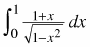2.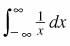3.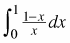4.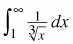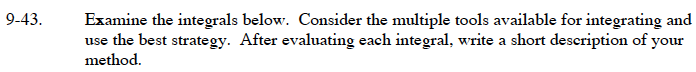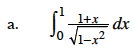$=\int_0^1\frac{1}{\sqrt{1-x^2}}dx+\int_0^1\frac{x}{\sqrt{1-x^2}}dx$

You should have the first integral committed to memory.
For the second integral, let u = 1 − x2.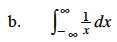This is an improper integral because the graph has a vertical asymptote at x = 0. Rewrite this integral using limits and two separate integrals before evaluating.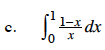$=\int_0^1\Big(\frac{1}{x}-1\Big)dx$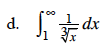Rewrite the integrand using exponents. This is also an improper integral.